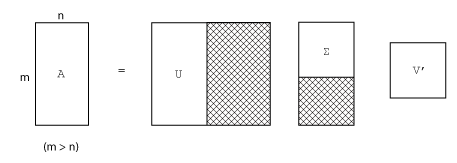## 奇异值

`$\begin{array}{l}Av=\sigma u\\ {A}^{H}u=\sigma v,\end{array}$`

`$\begin{array}{l}AV=U\Sigma \\ {A}^{H}U=V\Sigma .\end{array}$`

`$A=U\Sigma {V}^{H}.$`

m×n 矩阵的完整奇异值分解涉及：

• m×m 矩阵 U

• m×n 矩阵 Σ

• n×n 矩阵 V```A = 9 4 6 8 2 7```

```[U,S,V] = svd(A) U = 0.6105 -0.7174 0.3355 0.6646 0.2336 -0.7098 0.4308 0.6563 0.6194 S = 14.9359 0 0 5.1883 0 0 V = 0.6925 -0.7214 0.7214 0.6925```

```[U,S,V] = svd(A,0) U = 0.6105 -0.7174 0.6646 0.2336 0.4308 0.6563 S = 14.9359 0 0 5.1883 V = 0.6925 -0.7214 0.7214 0.6925```

### 低秩 SVD 逼近

`svds`使用 `svds` 计算 SVD 的 k 秩逼近。您可以指定一部分奇异值应为最大值、最小值还是最接近特定数字的值。`svds` 通常计算最可能的 k 秩逼近。
`svdsketch`使用 `svdsketch` 计算输入矩阵的满足指定容差的部分 SVD。`svds` 要求您指定秩，而 `svdsketch` 根据指定的容差以自适应方式确定矩阵草图的秩。`svdsketch` 最终使用的 k 秩逼近满足容差，但不同于 `svds`，它无法保证是最佳的。

```n = 1000; A = sprand(n,n,0.3);```

```S = svds(A) S = 130.2184 16.4358 16.4119 16.3688 16.3242 16.2838```

```S = svds(A,6,'smallest') S = 0.0740 0.0574 0.0388 0.0282 0.0131 0.0066```